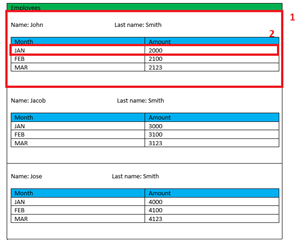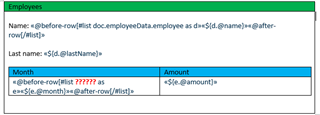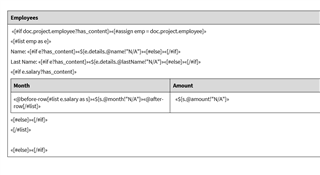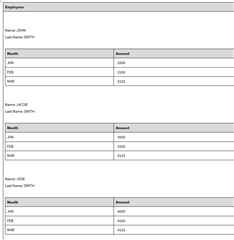# Nested loops inside the DOCX template with XML as data source

What's the best practice for this use case?

I have XML with the data similar to this:

```<employeeData>
<employee name="JOHN" lastName="SMITH">
<salary month="JAN" amount="2000" />
<salary name="FEB" amount="2100" />
<salary name="MAR" amount="2123" />
</employee>
<employee name="JACOB" lastName="SMITH">
<salary month="JAN" amount="3000" />
<salary name="FEB" amount="3100" />
<salary name="MAR" amount="3123" />
</employee>
<employee name="JOSE" lastName="SMITH">
<salary month="JAN" amount="4000" />
<salary name="FEB" amount="4100" />
<salary name="MAR" amount="4123" />
</employee>
</employeeData>```

In DOCX document i would like to achieve this:So, how can I configure my template to loop inside the loop?

I believe it would be something like this:But I'm missing this code for looping inside each employee tag.

Discussion posts and replies are publicly visible

Parents
• Hi ,

Working with complex structure XML (Multi level), in freemarker can be trick but you can apply some workarounds.

To implement your scenario I changed a little bit your input XML as well as your template. Please see the format that I used in my implementation:

```<project>
<employee >
<details name="JOHN" lastName="SMITH" />
<salary month="JAN" amount="2000" />
<salary month="FEB" amount="2100" />
<salary month="MAR" amount="2123" />
</employee>
<employee >
<details name="JACOB" lastName="SMITH" />
<salary month="JAN" amount="3000" />
<salary month="FEB" amount="3100" />
<salary month="MAR" amount="3123" />
</employee>
<employee >
<details name="JOSE" lastName="SMITH" />
<salary month="JAN" amount="4000" />
<salary month="FEB" amount="4100" />
<salary month="MAR" amount="4123" />
</employee>
</project>```

The template was configured like this:The result document is:Hope that helps

Acacio B.

• Hi ,

Working with complex structure XML (Multi level), in freemarker can be trick but you can apply some workarounds.

To implement your scenario I changed a little bit your input XML as well as your template. Please see the format that I used in my implementation:

```<project>
<employee >
<details name="JOHN" lastName="SMITH" />
<salary month="JAN" amount="2000" />
<salary month="FEB" amount="2100" />
<salary month="MAR" amount="2123" />
</employee>
<employee >
<details name="JACOB" lastName="SMITH" />
<salary month="JAN" amount="3000" />
<salary month="FEB" amount="3100" />
<salary month="MAR" amount="3123" />
</employee>
<employee >
<details name="JOSE" lastName="SMITH" />
<salary month="JAN" amount="4000" />
<salary month="FEB" amount="4100" />
<salary month="MAR" amount="4123" />
</employee>
</project>```

The template was configured like this:The result document is:Hope that helps

Acacio B.

Children
No Data